Home | | Maths 12th Std | Exercise 4.5: Inverse Trigonometric Functions

# Exercise 4.5: Inverse Trigonometric Functions

Maths Book back answers and solution for Exercise questions - Mathematics : Inverse Trigonometric Functions: Exercise Questions with Answer, Solution

EXERCISE 4.5

1. Find the value, if it exists. If not, give the reason for non-existence.2. Find the value of the expression in terms of , with the help of a reference triangle.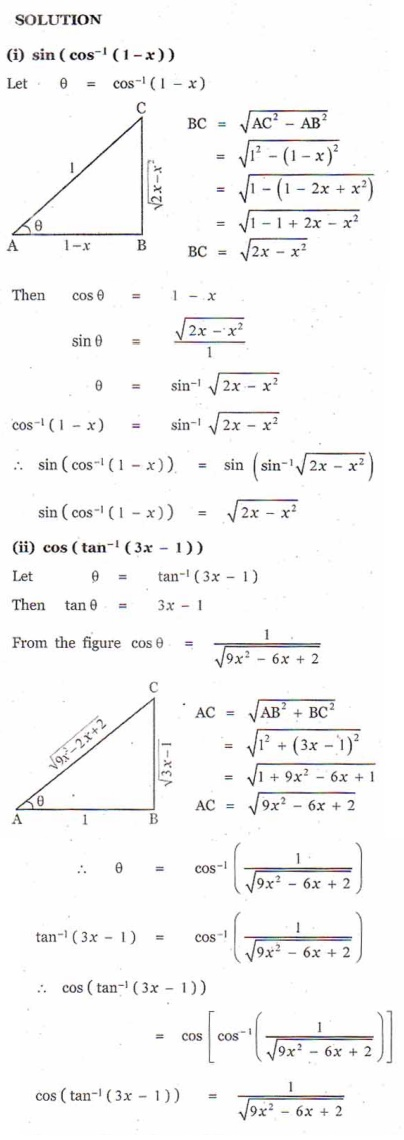3. Find the value of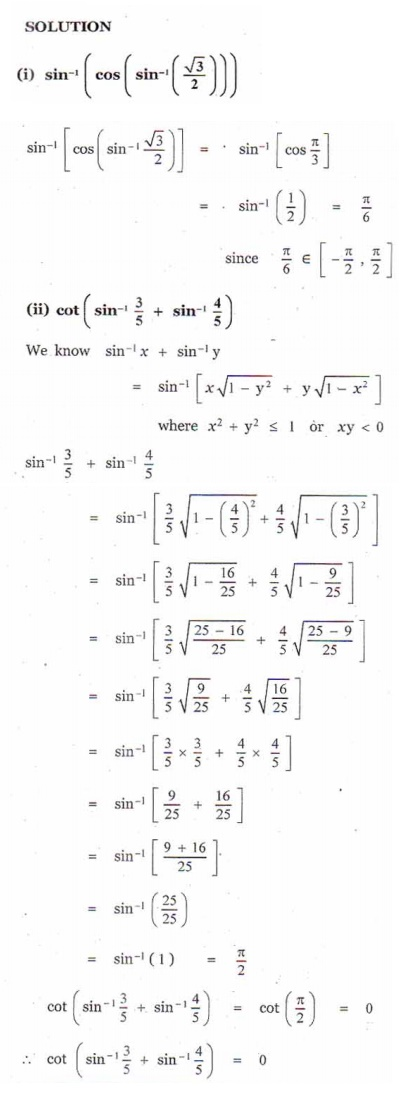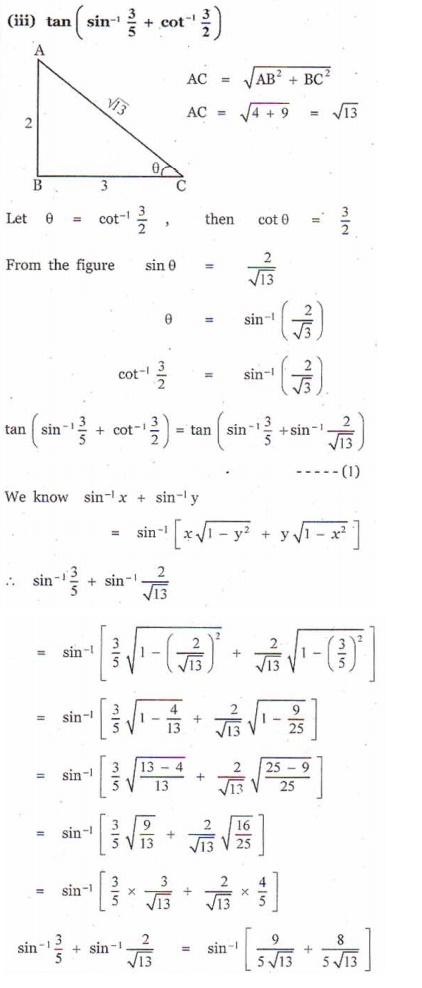4.Prove that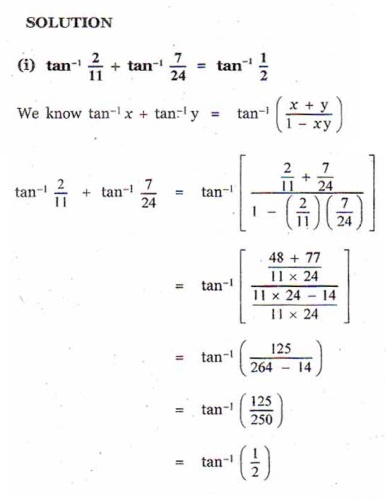5.Prove that tan+ tan+ tan= tan1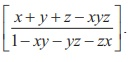6. If  tan1  + tan1  + tan1  = π , show that + + = xyz .7. Prove that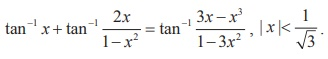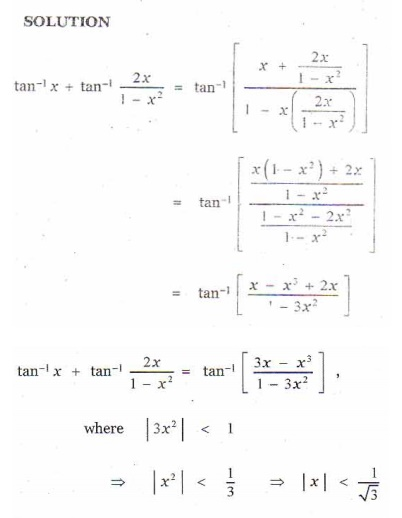8.Simplify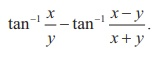9. Solve: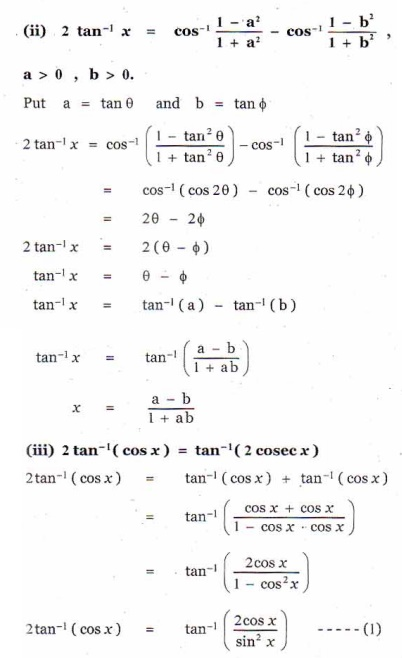10. Find the number of solution of the equation tan-1 ( x -1) + tan-1 x + tan-1 ( x +1) = tan-1 (3x).